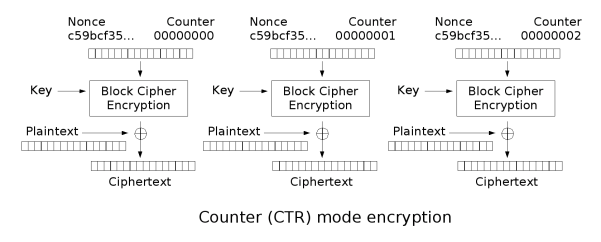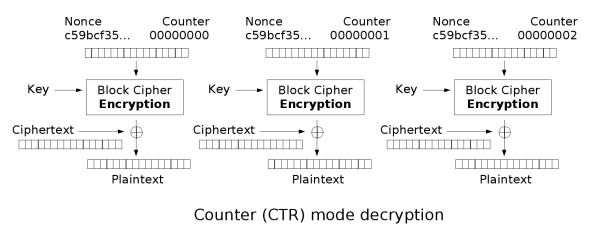# 密码学基础学习总结-07

## 前面说的Key的问题

wiki有言：

AES（Rijndael）使用一个密钥生成方案把一个短密钥扩展成各轮用于加密的子密钥。该方案的名称就叫做Rijndael密钥生成方案（Rijndael key schedule）。对于不同位数的原始密钥(128, 192, 256)，加密的轮次是不相同的，但每一轮都需要与128位的密钥异或，而根据原始密钥生成各轮子密钥的过程就是由Rijndael密钥生成方案完成的。

### RotWord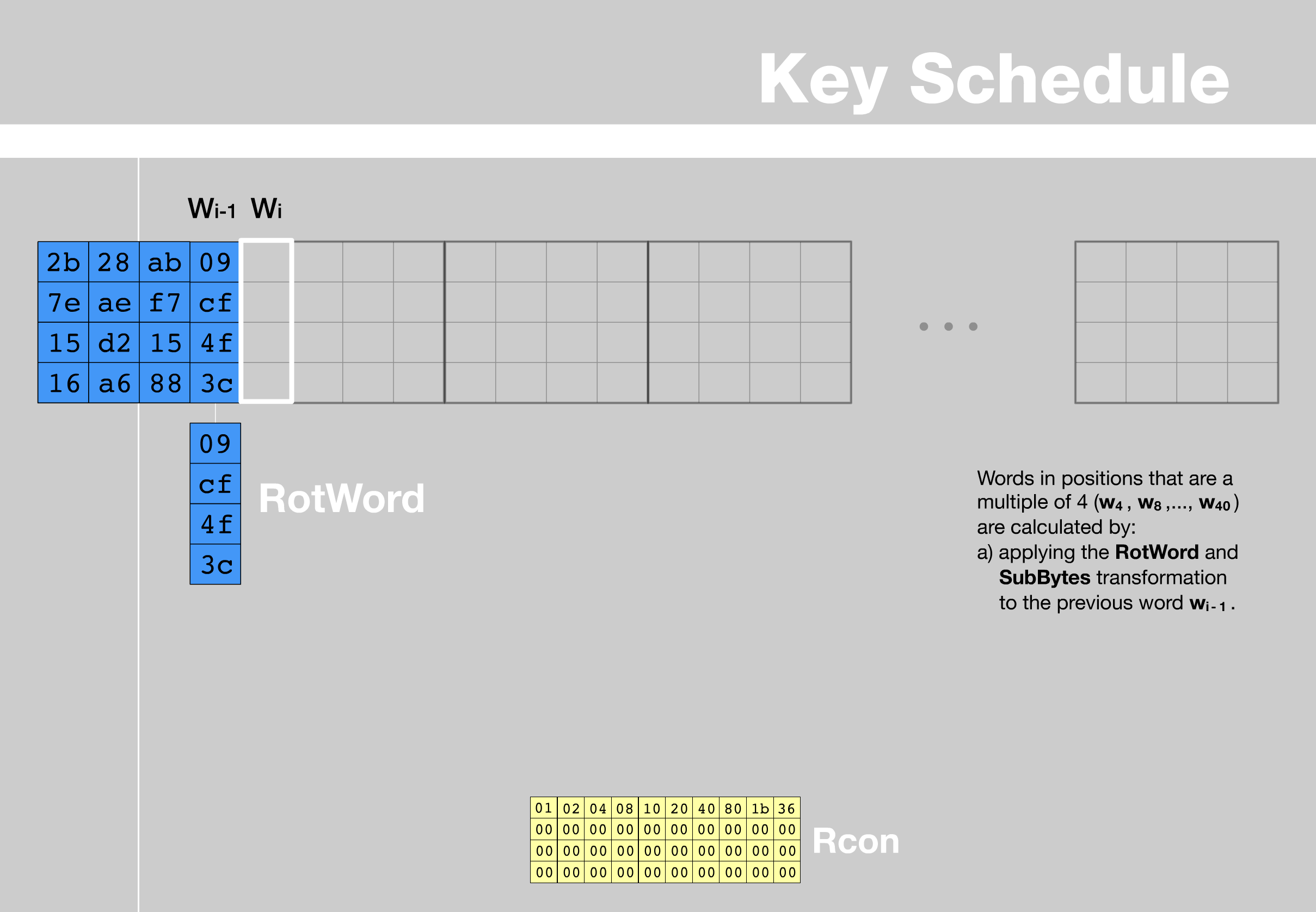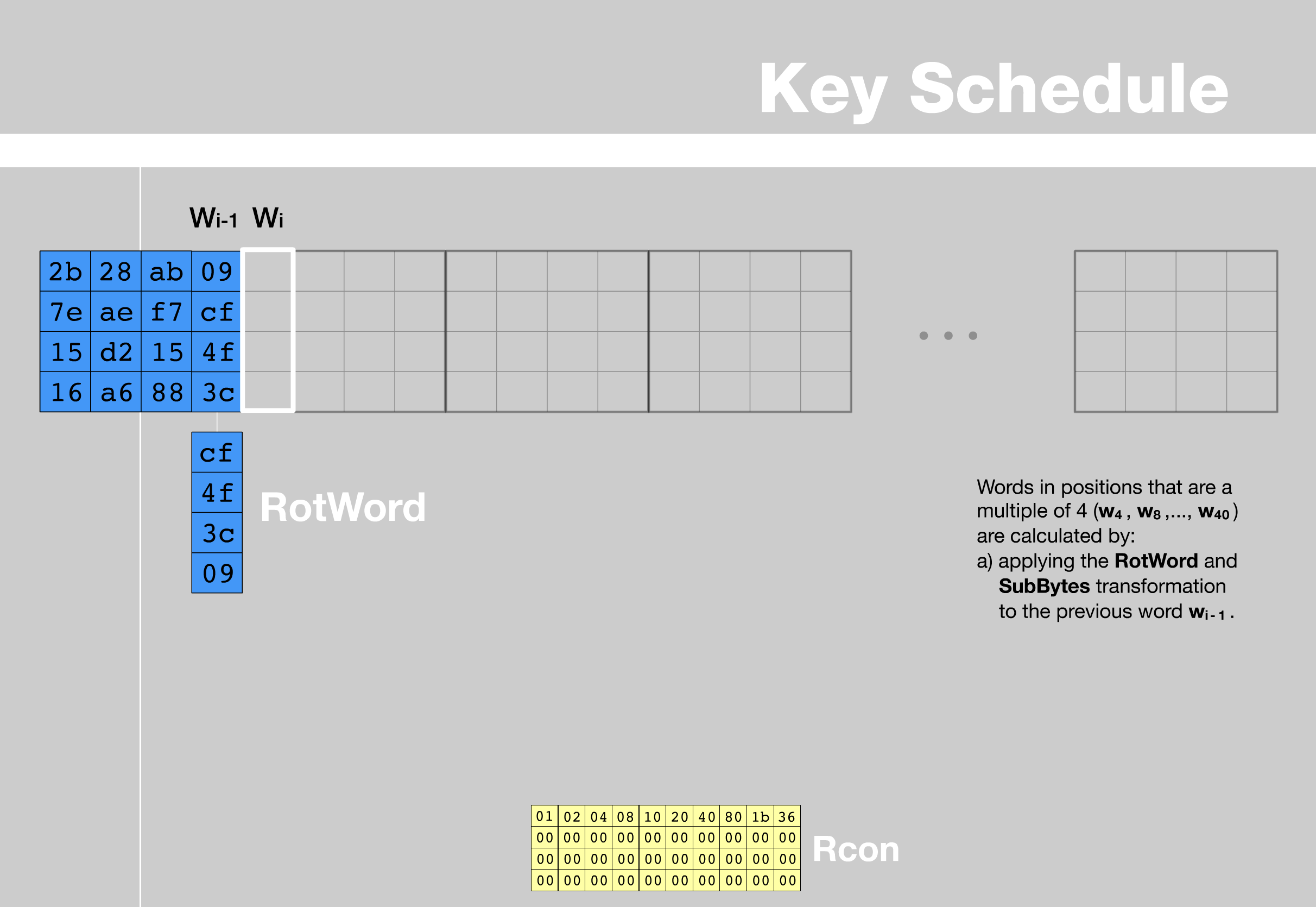### 替换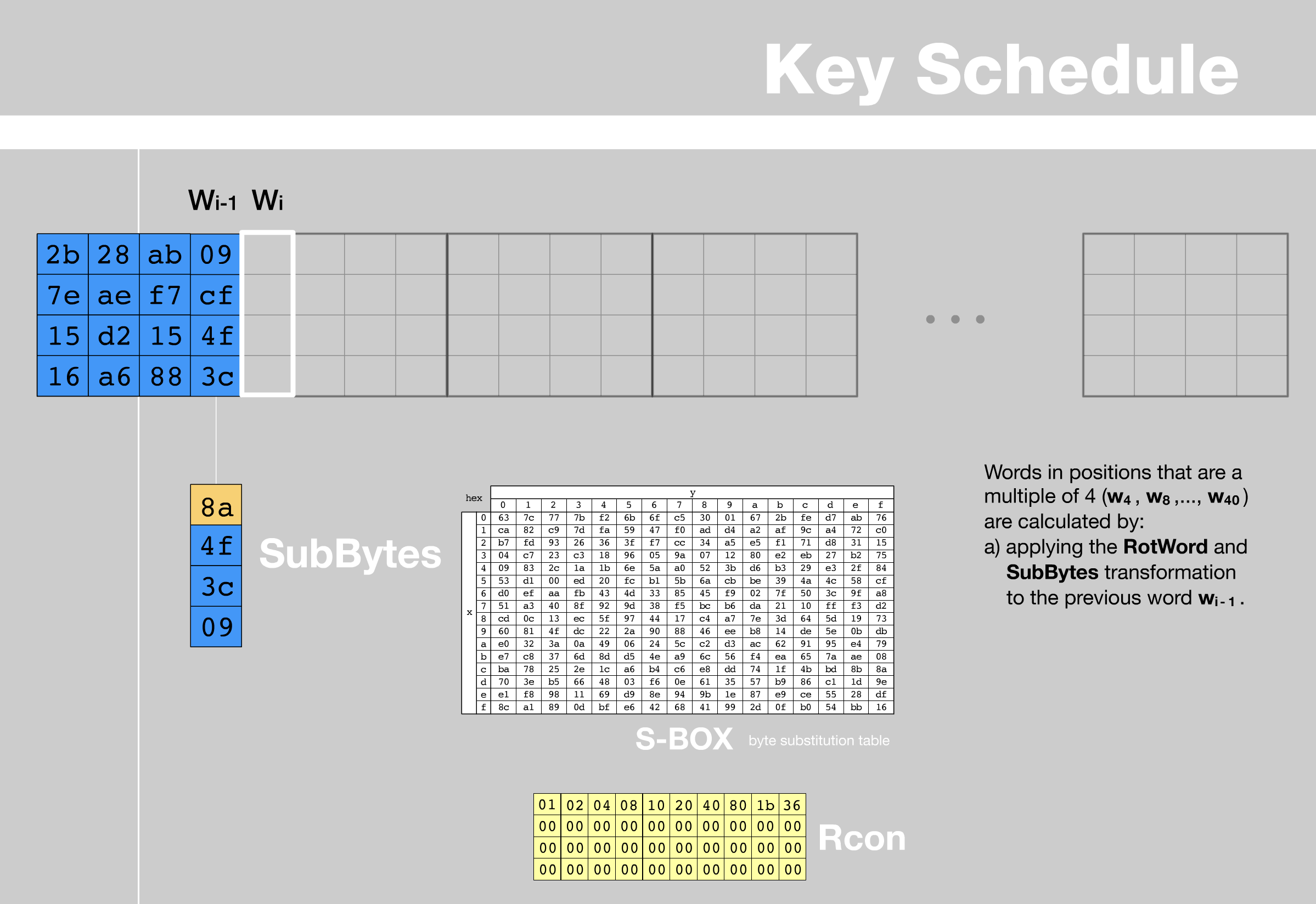### 求2的幂次操作(Rcon)

wiki有言：

Rcon在Rijndael的参考文档里称为求2的幂次。需要注意的是这个操作不是作用在常规的整数域中的，它是作用在Rijndael有限域中的。2的多项式形式为

$2=00000010=0x^7+0x^6+0x^5+0x^4+0x^3+0x^2+1x+0=b$，计算的公式为：

$rcon(i)=2^{i-1}=2*2^{-2}=2*rcon(i-1)$

$rcon(i)=b^{i-1}mod(x^8+x^4+x^3+x+1)$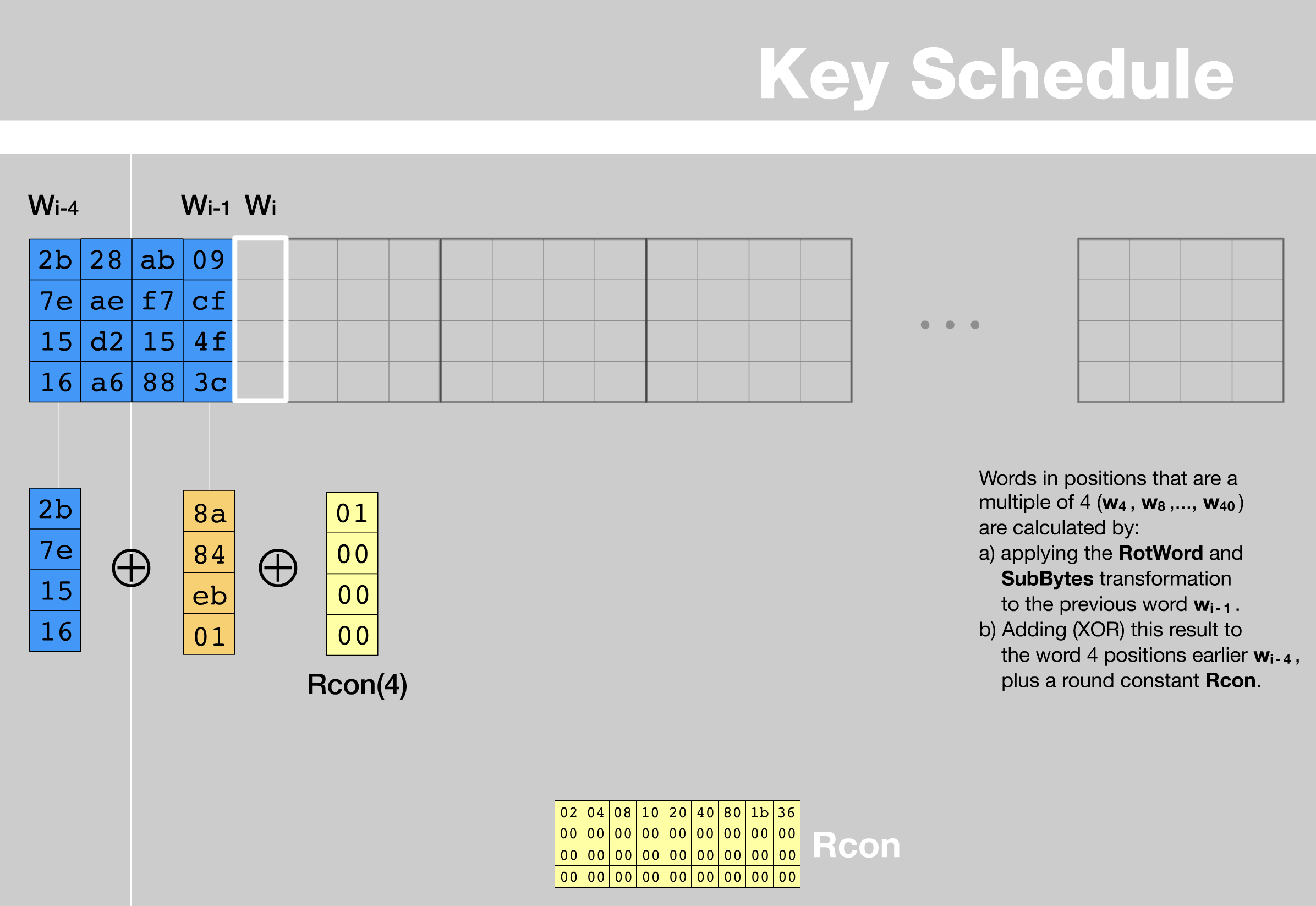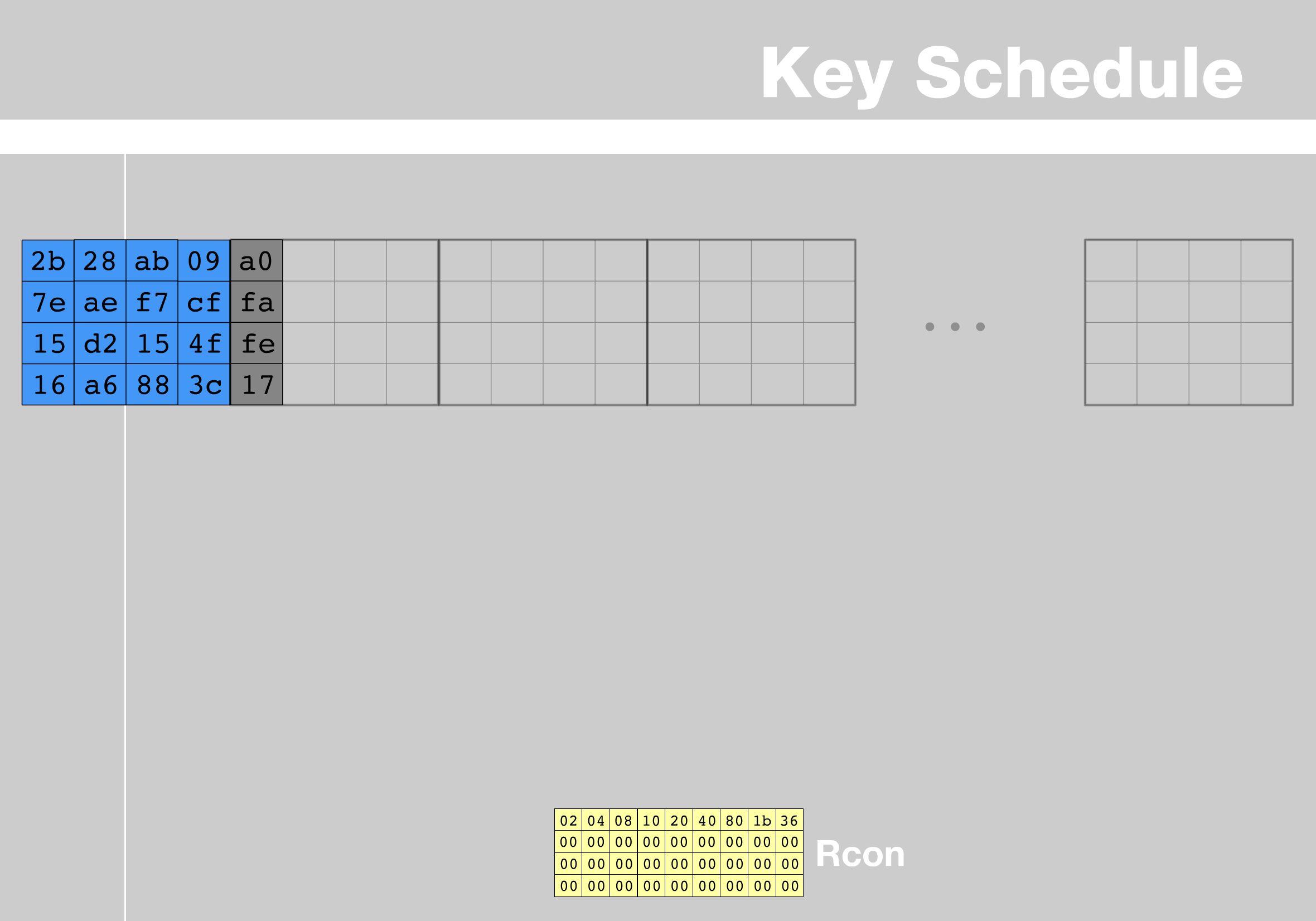## 分组密码的工作方式

### 常用模式

#### ECB 电子密码本模式 （其实不常用，因为不安全）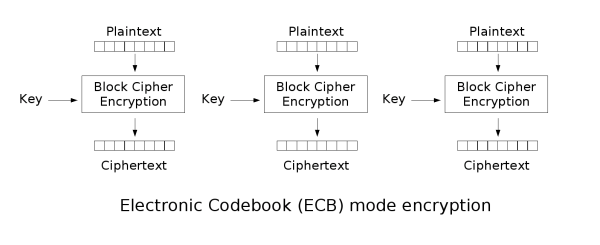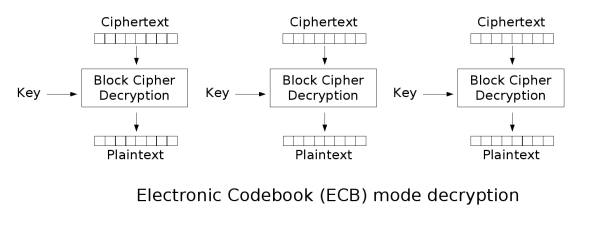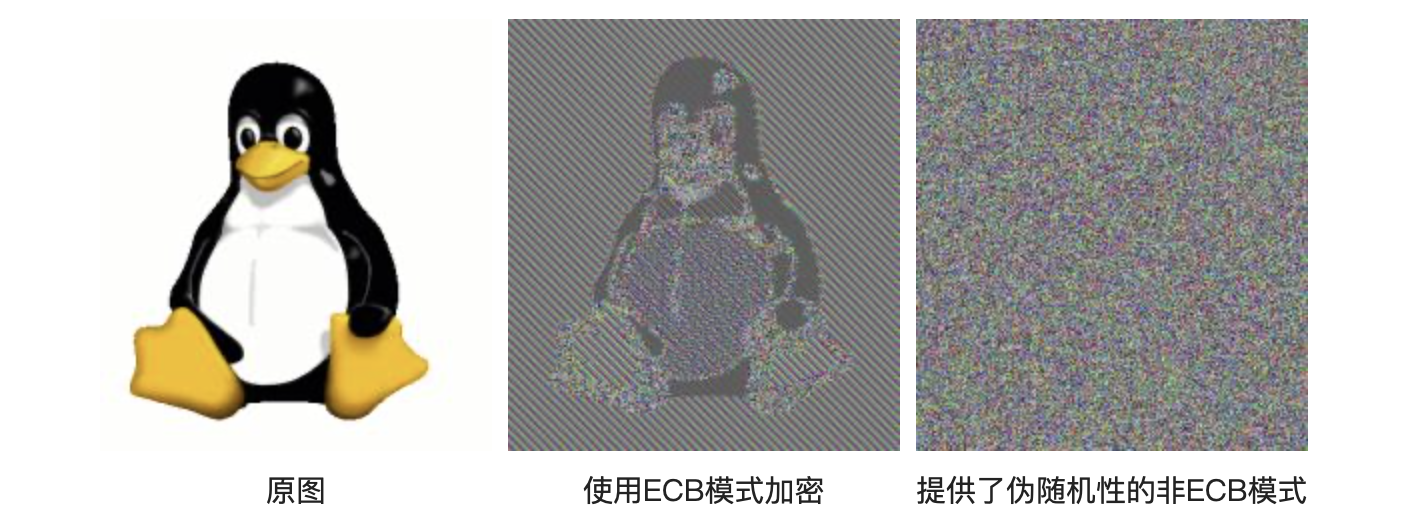#### CBC 密码块链接模式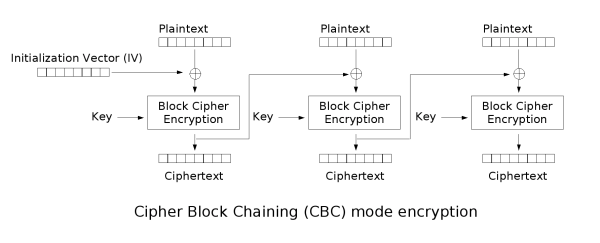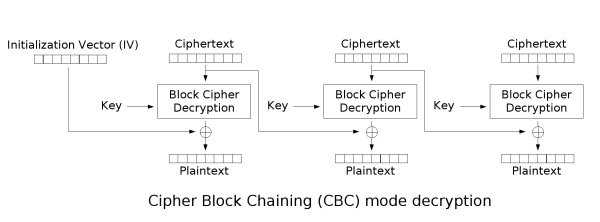• 加密：$C_i = Enc_k(p_i \oplus C_{i-1});C_0 = IV$
• 解密：$P_{i} = Dec_{k}(C_i)\oplus C_{i-1};C_{0} = IV$

#### CFB 密文反馈模式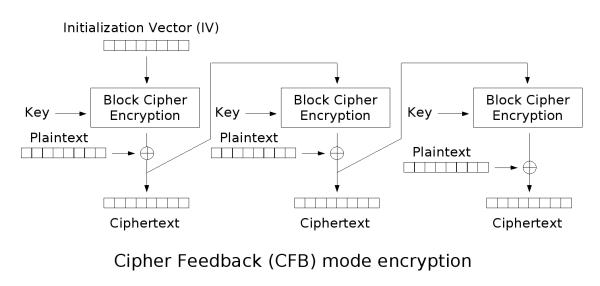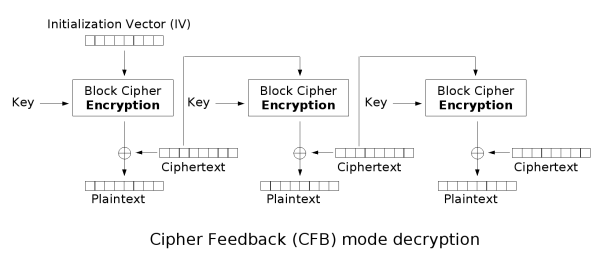CFB和CBC实际上还蛮相似的，图中描写的很详细，下面还是给出一个公式来描述加密和解密流程：

• 加密：$C_i=Enc_{k}(C_{i-1}) \oplus P_{1};C_0 = IV$
• 解密：$P_i = Enc_{k}(C_{i-1})\oplus C_{i};C_0 = IV$

#### OFB 输出反馈模式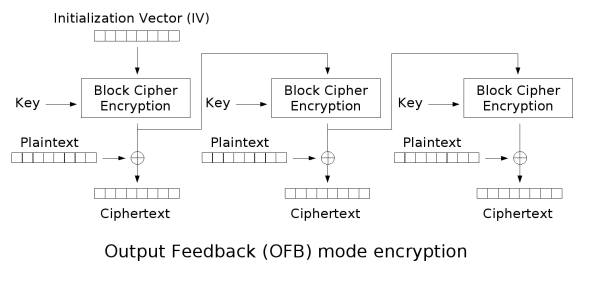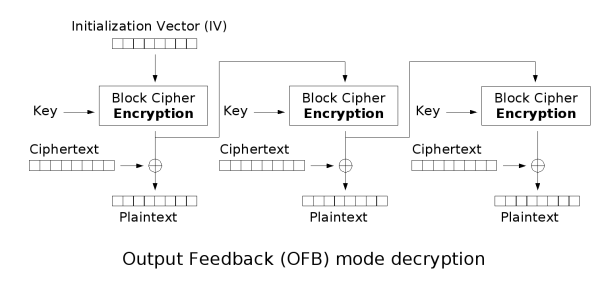#### CTR 计数器模式